CAT  >  Test: CAT Quant 2019 (Shift - 2)

# Test: CAT Quant 2019 (Shift - 2)

Test Description

## 34 Questions MCQ Test | Test: CAT Quant 2019 (Shift - 2)

Test: CAT Quant 2019 (Shift - 2) for CAT 2023 is part of CAT preparation. The Test: CAT Quant 2019 (Shift - 2) questions and answers have been prepared according to the CAT exam syllabus.The Test: CAT Quant 2019 (Shift - 2) MCQs are made for CAT 2023 Exam. Find important definitions, questions, notes, meanings, examples, exercises, MCQs and online tests for Test: CAT Quant 2019 (Shift - 2) below.
Solutions of Test: CAT Quant 2019 (Shift - 2) questions in English are available as part of our course for CAT & Test: CAT Quant 2019 (Shift - 2) solutions in Hindi for CAT course. Download more important topics, notes, lectures and mock test series for CAT Exam by signing up for free. Attempt Test: CAT Quant 2019 (Shift - 2) | 34 questions in 60 minutes | Mock test for CAT preparation | Free important questions MCQ to study for CAT Exam | Download free PDF with solutions
 1 Crore+ students have signed up on EduRev. Have you?
Test: CAT Quant 2019 (Shift - 2) - Question 1

### The average of 30 integers is 5. Among these 30 integers, there are exactly 20 which do not exceed 5. What is the highest possible value of the average of these 20 integers?

Detailed Solution for Test: CAT Quant 2019 (Shift - 2) - Question 1 It is given that the average of the 30 integers = 5

Sum of the 30 integers = 30*5=150

There are exactly 20 integers whose value is less than 5.

To maximise the average of the 20-integers, we have to assign minimum value to each of the remaining 10 integers

so the sum of 10 integers = 10*6=60

The sum of the 20 integers = 150-60 = 90

Average of 20 integers = 90/20=4.5

Test: CAT Quant 2019 (Shift - 2) - Question 2

### Amal invests Rs 12000 at 8% interest, compounded annually, and Rs 10000 at 6% interest, compounded semi-annually, both investments being for one year. Bimal invests his money at 7.5% simple interest for one year. If Amal and Bimal get the same amount of interest, then the amount, in Rupees, invested by Bimal is

Detailed Solution for Test: CAT Quant 2019 (Shift - 2) - Question 2 The amount with Amal at the end of 1 year = 12000*1.08+10000*1.03*1.03=23569

Interest received by Amal = 23569 – 22000=1569

Let the amount invested by Bimal = 100b

Interest received by Bimal = 100b*7.5*1/100=7.5b

It is given that the amount of interest received by both of them is the same

7.5b=1569

b=209.2

Amount invested by Bimal = 100b = 20920

Test: CAT Quant 2019 (Shift - 2) - Question 3

### What is the largest positive integer n such that n2+7n+12/n2 – n-12 is also a positive integer?

Detailed Solution for Test: CAT Quant 2019 (Shift - 2) - Question 3 n2+3n+4n+12/n2 - 4n+3n - 12

= n (n+3)+4(n+3)/n(n-4)+3(n-4)

= (n+4)(n+3)/(n-4)(n+3)

= (n+4)/(n-4)

=(n-4)+8/(n-4)

= 1 + 8/(n-4) which will be maximum when n-4 =8

n=12

Test: CAT Quant 2019 (Shift - 2) - Question 4

How many pairs (m, n) of positive integers satisfy the equation m2 + 105 = n2?

Detailed Solution for Test: CAT Quant 2019 (Shift - 2) - Question 4 n2- m2 = 105

(n-m) (n+m) = 1 *105, 3*35, 5*21, 7*15, 15*7, 21 *5, 35*3,105*1.

n – m=1, n+m=105==> n=53, m=52

n – m=3, n+m=35 ==> n=19, m=16

n – m=5, n+m=21 ==>n=13, m=8

n – m=7, n+m=15 ==> n=11, m=4

n – m=15, n+m=7 ==> n=11, m=-4

n – m=21, n+m=5 ==> n=13, m=-8

n – m=35, n+m=3 ==> n=19, m=-16

n – m=105, n+m=1 ==> n=53, m=-52

Since only positive integer values of m and n are required. There are 4 possible solutions.

Test: CAT Quant 2019 (Shift - 2) - Question 5

Two ants A and B start from a point P on a circle at the same time, with A moving clock-wise and B moving anti-clockwise. They meet for the first time at 10:00 am when A has covered 60% of the track. If A returns to P at 10:12 am, then B returns to P at

Detailed Solution for Test: CAT Quant 2019 (Shift - 2) - Question 5 When A and B met for the first time at 10:00 AM, A covered 60% of the track.

So B must have covered 40% of the track.

It is given that A returns to P at 10:12 AM i.e. A covers 40% of the track in 12 minutes 60% of the track in 18 minutes

B covers 40% of track when A covers 60% of the track.

B covers 40% of the track in 18 minutes.

B will cover the rest 60% in 27 minutes, hence it will return to B at 10:27 AM

Test: CAT Quant 2019 (Shift - 2) - Question 6

Let a1, a2... be integers such that

a1- a2 + a3-a4+.... + (—1) n-1an = n, for all n ≥ 1.

Then a51 + a52+ ....+a1023 equals

Detailed Solution for Test: CAT Quant 2019 (Shift - 2) - Question 6 a1 − a2 + a3 − a4+.... + (−1) n−1an = n

It is clear from the above equation that when n is odd, the co-efficient of a is positive otherwise negative.

a1- a2 = 2

a1 = a2 + 2

a1 − a2 + a3 = 3

On substituting the value of ai in the above equation, we get

a3 = 1

a1 -a2 + a3 -a4 = 4

On substituting the values of a1, a3 in the above equation, we get

a4 = -1

a1 – a2 + a3 – a4 + a5 = 5

On substituting the values of a1, a3, a4in the above equation, we get

a5 = 1

So we can conclude that a3, a5,a7….. an+1 = 1 and a2, a4, a6....a2n = -1

Now we have to find the value of a51 + a52 + ....+ a1023

Number of terms = 1023=51+(n-1)1

n=973

There will be 486 even and 487 odd terms, so the value of a51 + a52 + .... + a1023 = 486*-1+487*1

Test: CAT Quant 2019 (Shift - 2) - Question 7

Two circles, each of radius 4 cm, touch externally. Each of these two circles is touched externally by a third circle. If these three circles have a common tangent, then the radius of the third circle, in cm, is

Detailed Solution for Test: CAT Quant 2019 (Shift - 2) - Question 7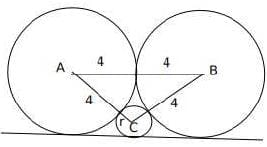Let 'h’ be the height of the triangle ABC, semi perimeter(S) = (4+4+r+4+4+r)/2 = 8 + r,

a=4 +r, b = 4 + r, c = 8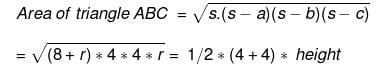Height (h) = √(8+r)r

Now, h + r = 4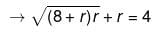(Considering the height of the triangle)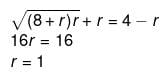Alternatively,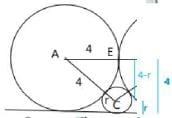AE2 + EC2 = AC2⟶ 42 + (4 − r)2 = (4 + r)2⟶⟶⟶ r = 1

Test: CAT Quant 2019 (Shift - 2) - Question 8

Let A be a real number. Then the roots of the equation x2- 4x - log2A = 0 are real and distinct if and only if

Detailed Solution for Test: CAT Quant 2019 (Shift - 2) - Question 8 The roots of x2- 4x - log2A = 0 will be real and distinct if and only if the discriminant is greater than zero

16+4*log2A > 0

log2A > -4

A> 1/16

Test: CAT Quant 2019 (Shift - 2) - Question 9

The quadratic equation x2 + bx + c = 0 has two roots 4a and 3a, where a is an integer. Which of the following is a possible value of b2 + c?

Detailed Solution for Test: CAT Quant 2019 (Shift - 2) - Question 9 Given, The quadratic equation x2 + bx + c = 0 has two roots 4a and 3a

7a=-b

12a2 = c

We have to find the value of b2 + c = 49a2+ 12a2=61a2

Now let’s verify the options

61 a2 = 3721 ==> a= 7.8 which is not an integer

61 a2 = 361 ==> a= 2.42 which is not an integer

61 a2 = 427 ==> a= 2.64 which is not an integer

61 a2 = 3721 ==> a= 3 which is an integer

Test: CAT Quant 2019 (Shift - 2) - Question 10

The base of a regular pyramid is a square and each of the other four sides is an equilateral triangle, length of each side being 20 cm. The vertical height of the pyramid, in cm, is

Detailed Solution for Test: CAT Quant 2019 (Shift - 2) - Question 10 It is given that the base of the pyramid is square and each of the four sides are equilateral triangles.

Length of each side of the equilateral triangle = 20cm

Since the side of the triangle will be common to the square as well, the side of the square = 20cm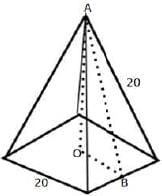Let h be the vertical height of the pyramid i.e. OA

OB = 10 since it is half the side of the square

AB is the height of the equilateral triangle i.e. 10√3

AOB is a right angle, so applying the Pythagorean formula, we get

OA2 + OB2 = AB2

h2 + 100= 300

h=10√2

Test: CAT Quant 2019 (Shift - 2) - Question 11

Let ABC be a right-angled triangle with hypotenuse BC of length 20 cm. If AP is perpendicular on BC, then the maximum possible length of AP, in cm, is

Detailed Solution for Test: CAT Quant 2019 (Shift - 2) - Question 11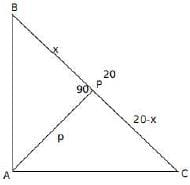Let p be the length of AP.

It is given that ∠BAC = 90 and ∠APC = 90

Let ∠ABC = ϴ, then ∠BAP= 90 - ϴ and ∠BCA =90 - ϴ

so ∠PAC = ϴ

Triangles BPA and APC are similar

p2 = x (20 - x)

we have to maximize the value of p, which will be maximum when x=20-x

x=10

Test: CAT Quant 2019 (Shift - 2) - Question 12

If x is a real number, then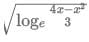is a real number if and only if

Detailed Solution for Test: CAT Quant 2019 (Shift - 2) - Question 12will be real if loge 4x-x2/3 ≥ 0

4x-x2/3>=1

4x - x2- 3 >= 0

x2 − 4x + 3 =< />

1=< x="" />< />

Test: CAT Quant 2019 (Shift - 2) - Question 13

If 5x – 3y= 13438 and 5x-1 + 3y+1 = 9686, then x + y equals

Detailed Solution for Test: CAT Quant 2019 (Shift - 2) - Question 13 5x– 3y = 13438 and 5x-1 + 3y+1 = 9686

5x + 3y * 15 = 9686 * 5

5x + 3y * 15 = 48430

16*3y =34992

3y = 2187

y = 7

5x = 13438+2187=15625

x=6

x+y = 13

Test: CAT Quant 2019 (Shift - 2) - Question 14

The strength of a salt solution is p% if 100 ml of the solution contains p grams of salt. Each of three vessels A,B, C contains 500ml of salt solution of strengths 10%, 22%, and 32%, respectively. Now, 100 ml of the solution in vessel A is transferred tovessel B.Then, 100 ml of the solution in vessel B is transferred to vessel C. Finally, 100 ml of the solution in vessel C is transferred to vessel A. The strength, in percentage, of the resulting solution in vessel A is

Detailed Solution for Test: CAT Quant 2019 (Shift - 2) - Question 14 Each of three vessels A, B, C contains 500 ml of salt solution of strengths 10%, 22%, and 32%, respectively.

The amount of salt in vessels A, B, C = 50 ml, 110 ml, 160 ml respectively.

The amount of water in vessels A, B, C = 450 ml, 390 ml, 340 ml respectively.

In 100 ml solution in vessel A, there will be 10ml of salt and 90 ml of water

Now, 100 ml of the solution in vessel A is transferred to vessel B. Then, 100 ml of the solution in vessel B is transferred to vessel C.

Finally, 100 ml of the solution in vessel C is transferred to vessel A

i.e. after the first transfer, the amount of salt in vessels A, B, C = 40,120,160 ml respectively,

after the second transfer, the amount of salt in vessels A, B, C =40,400,180 ml respectively.

After the third transfer, the amount of salt in vessels A, B, C =70,100,150 respectively.

Each transfer can be captured through the following table.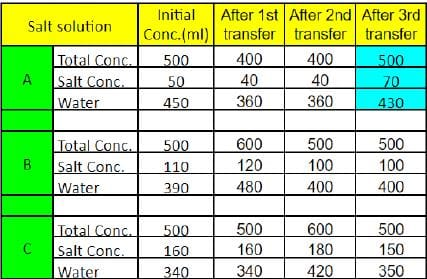Percentage of salt in vessel A = 70/500 x 100 =14%

Test: CAT Quant 2019 (Shift - 2) - Question 15

A cyclist leaves A at 10 am and reaches B at 11 am. Starting from 10:01 am, every minute a motorcycle leaves A and moves towards B. Forty-five such motorcycles reach B by 11 am. All motorcycles have the same speed. If the cyclist had doubled his speed, how many motorcycles would have reached B by the time the cyclist reached B?

Detailed Solution for Test: CAT Quant 2019 (Shift - 2) - Question 15 It is given that starting from 10:01 am, every minute a motorcycle leaves A and moves towards B.

Forty-five such motorcycles reach B by 11 am.

It means that the forty-fifth motorcycle starts at 10:45 AM at A and reaches B by 11:00 AM i.e., 15 minutes.

Since the speed of all the motorcycles is the same, all the motorcycles will take the same duration i.e. 15 minutes.

If the cyclist doubles the speed, then he will reach B by 10:30 AM. (Since if the speed is doubled, time is reduced by half)

Since each motorcycle takes 15 minutes to reach B, 15 motorcycles would have reached B by the time the cyclist reaches B

Test: CAT Quant 2019 (Shift - 2) - Question 16

A man makes complete use of 405 cc of iron, 783 cc of aluminium, and 351 cc of copper to make a number of solid right circular cylinders of each type of metal. These cylinders have the same volume and each of these has radius 3 cm. If the total number of cylinders is to be kept at a minimum, then the total surface area of all these cylinders, in sq cm, is

Detailed Solution for Test: CAT Quant 2019 (Shift - 2) - Question 16 It is given that the volume of all the cylinders is the same, so the volume of each cylinder = HCF of (405,783, 351) = 27

The number of iron cylinders = 405/27 = 15

The number of aluminium cylinders = 783/27 = 29

The number of copper cylinders = 315/27 = 13

15*πr2h= 405

15*π9*h= 405

πh = 3

Now we have to calculate the total surface area of all the cylinders

Total number of cylinders = 15+29+13 = 57

Total surface area of the cylinder = 57*(2πrh + 2πr2)

=57(2*3*3 + 2*9*π)

=1026(1 + π)

Test: CAT Quant 2019 (Shift - 2) - Question 17

The real root of the equation 26x + 23x+2- 21 = 0 is

Detailed Solution for Test: CAT Quant 2019 (Shift - 2) - Question 17 Let 23x = v

26x + 23x+2 -21 = 0

= v2 + 4v - 21 =0

=(v+7)(v-3)=0

v = 3,-7

23x = 3 or 23x = -7(This can be negated)

3x=log23

x=log23/3

Test: CAT Quant 2019 (Shift - 2) - Question 18

How many factors of 24 x 35 x 104 are perfect squares which are greater than 1?

Detailed Solution for Test: CAT Quant 2019 (Shift - 2) - Question 18 24 x 35 x 104

=24 x 35 x 24 * 54

=28 x 35 x 54

For the factor to be a perfect square, the factor should be even power of the number.

In 28, the factors which are perfect squares are 20, 22, 24, 26, 28 = 5

Similarly, in 35, the factors which are perfect squares are 30, 32,34 = 3

In 54, the factors which are perfect squares are 50, 52, 54 = 3

Number of perfect squares greater than 1 = 5*3*3-1

=44

Test: CAT Quant 2019 (Shift - 2) - Question 19

In a six-digit number, the sixth, that is, the rightmost, digit is the sum of the first three digits, the fifth digit is the sum of first two digits, the third digit is equal to the first digit, the second digit is twice the first digit and the fourth digit is the sum of fifth and sixth digits. Then, the largest possible value of the fourth digit is

Detailed Solution for Test: CAT Quant 2019 (Shift - 2) - Question 19 Let the six-digit number be ABCDEF

F = A+B+C, E= A+B, C=A, B= 2A, D= E+F.

Therefore D = 2A+2B+C = 2A + 4A + A= 7A.

A cannot be 0 as the number is a 6-digit number.

A cannot be 2 as D would become 2 digit number.

Therefore A is 1 and D is 7.

Test: CAT Quant 2019 (Shift - 2) - Question 20

John jogs on track A at 6 kmph and Mary jogs on track B at 7.5 kmph. The total length of tracks A and B is 325 metres. While John makes 9 rounds of track A, Mary makes 5 rounds of track B. In how many seconds will Mary make one round of track A?

Detailed Solution for Test: CAT Quant 2019 (Shift - 2) - Question 20 Speed of John = 6kmph

Speed of Mary = 7.5 kmph

Lengths of tracks A and B = 325m

Let the length of track A be a, then the length of track B = 325-a

9 rounds of John on track A = 5 rounds of Mary on track B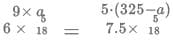On solving we get, 13a=1300

a = 100

The length of track A = 100m, track B = 225m

Mary makes one round of track A =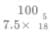= 48 sec

Test: CAT Quant 2019 (Shift - 2) - Question 21

In 2010, a library contained a total of 11500 books in two categories - fiction and nonfiction. In 2015, the library contained a total of 12760 books in these two categories. During this period, there was 10% increase in the fiction category while there was 12% increase in the non-fiction category. How many fiction books were in the library in 2015?

Detailed Solution for Test: CAT Quant 2019 (Shift - 2) - Question 21 Let the number of fiction and non-fiction books in 2010 = 100a, 100b respectively

It is given that the total number of books in 2010 = 11500

100a+100b = 11500……… Eq 1

The number of fiction and non-fiction books in 2015 = 110a, 112b respectively

110a+112b = 12760…….. Eq2

On solving both the equations we get, b=55, a= 60

The number of fiction books in 2015 = 110*60=6600

Test: CAT Quant 2019 (Shift - 2) - Question 22

John gets Rs 57 per hour of regular work and Rs 114 per hour of overtime work. He works altogether 172 hours and his income from overtime hours is 15% of his income from regular hours. Then, for how many hours did he work overtime?

Detailed Solution for Test: CAT Quant 2019 (Shift - 2) - Question 22 It is given that John works altogether 172 hours i.e. including regular and overtime hours.

Let a be the regular hours, 172-a will be the overtime hours

John's income from regular hours = 57*a

John's income for working overtime hours = (172-a)*144

It is given that his income from overtime hours is 15% of his income from regular hours

a*57*0.15 = (172-a)*114

a=160

The number of hours for which he worked overtime = 172 – 160=12 hrs

Test: CAT Quant 2019 (Shift - 2) - Question 23

If (2n + 1) + (2n + 3) + (2n + 5) + ... + (2n + 47) = 5280, then what is the value of 1 + 2 + 3 +... + n?

Detailed Solution for Test: CAT Quant 2019 (Shift - 2) - Question 23 Let us first find the number of terms

47=1+ (n-1)2

n=24

24*2n+1+3+5+....47=5280

48n+576=5280

48n=4704

n=98

Sum of first 98 terms = 98*99/2

=4851

Positive Mark: 3

Negative Mark: 0

Test: CAT Quant 2019 (Shift - 2) - Question 24

A shopkeeper sells two tables, each procured at cost price p, to Amal and Asim at a profit of 20% and at a loss of 20%, respectively. Amal sells his table to Bimal at a profit of 30%, while Asim sells his table to Barun at a loss of 30%. If the amounts paid by Bimal and Barun are x and y, respectively, then (x - y) / p equals

Detailed Solution for Test: CAT Quant 2019 (Shift - 2) - Question 24 CP of the table at which the shopkeeper procured each table = p

It is given that shopkeeper sold the tables to Amal and Asim at a profit of 20% and at a loss of 20%, respectively

The selling price of the tables = 1.2p and 0.8p to Amal and Asim respectively.

Amal sells his table to Bimal at a profit of 30%

So, CP of the table by Bimal (x)= 1.2p*1.3 = 1.56p

Asim sells his table to Barun at a loss of 30%

So, CP of the table by Barun (y)= 0.7*0.8p = 0.56p

(x-y)/p = (1.56p-0.56p)/p = p/p=1

Test: CAT Quant 2019 (Shift - 2) - Question 25

In a triangle ABC, medians AD and BE are perpendicular to each other, and have lengths 12 cm and 9 cm, respectively. Then, the area of triangle ABC, in sq cm, is

Detailed Solution for Test: CAT Quant 2019 (Shift - 2) - Question 25 It is given that AD and BE are medians which are perpendicular to each other.

The lengths of AD and BE are 12cm and 9cm respectively.

It is known that the centroid G divides the median in the ratio of 2:1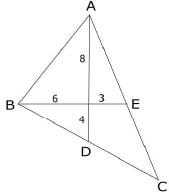Area of ΔABC = 2* Area of the triangle ABD

Area of ΔABD = Area of ΔAGB + Area of ΔBGD

Since ∠AGB = ∠BGD, 90 (Given)

Area of ΔAGB = ½ x 8 x 6 = 24

Area of ΔBGD = 1/2 x 6 x 4 = 12

Area of ΔABD = 24+12=36

Area of ΔABC = 2 x 36 = 72

Test: CAT Quant 2019 (Shift - 2) - Question 26

The number of common terms in the two sequences: 15, 19, 23, 27… 415 and 14, 19, 24, 29… 464 is

Detailed Solution for Test: CAT Quant 2019 (Shift - 2) - Question 26 A: 15,19,23,27,……., 415

B:14,19, 24, 29……, 464

Here the first common term = 19

Common difference = LCM of 5,4=20

19+(n-1)20 ≤ 415

(n-1)20 ≤ 396

(n-1) ≤ 19.8

n=20

Positive Mark: 3

Negative Mark: 0

Test: CAT Quant 2019 (Shift - 2) - Question 27

Let a, b, x, y be real numbers such that a2 + b2 = 25, x2 + y2 = 169, and ax + by = 65. If k = ay - bx, then

Detailed Solution for Test: CAT Quant 2019 (Shift - 2) - Question 27 (ax + by) 2 = 652

a2x2 + b2y2 + 2 abxy = 652

k = ay — bx

k2 = a2y2 + b2x2- 2abxy

(a2 + b2)(x2 + y2) = 25 * 169

a2x2 + a2y2 + b2x2 + b2y2 = 25 x 169

k2 = 652 - (25 x 169)

k = 0

Test: CAT Quant 2019 (Shift - 2) - Question 28

Mukesh purchased 10 bicycles in 2017, all at the same price. He sold six of these at a profit of 25% and the remaining four at a loss of 25%. If he made a total profit of Rs. 2000, then his purchase price of a bicycle, in Rupees, was

Detailed Solution for Test: CAT Quant 2019 (Shift - 2) - Question 28 Let the cost of each bicycle= 100b

CP of 10 bicycles = 1000b

It is given that he sold six of these at a profit of 25% and the remaining four at a loss of 25%

SP of 10 bicycles = 125b*6+75b*4

=1050b

Profit = 1050b-1000b =50b

50b=2000

CP = 100b = 4000

Test: CAT Quant 2019 (Shift - 2) - Question 29

In an examination, the score of A was 10% less than that of B, the score of B was 25% more than that of C, and the score of C was 20% less than that of D. If A scored 72, then the score of D was

Detailed Solution for Test: CAT Quant 2019 (Shift - 2) - Question 29 Let the score of D = 100d

The score of C = 20% less than that of D = 80d

The score of B = 25% more than C = 100d

The score of A = 1.0% less than B =90d

90d=72

100d= 72*100/90

Test: CAT Quant 2019 (Shift - 2) - Question 30

The salaries of Ramesh, Ganesh and Rajesh were in the ratio 6:5:7 in 2010, and in the ratio 3:4:3 in 2015. If Ramesh's salary increased by 25% during 2010-2015, then the percentage increase in Rajesh's salary during this period is closest to

Detailed Solution for Test: CAT Quant 2019 (Shift - 2) - Question 30 Let the salaries of Ramesh, Ganesh and Rajesh in 2010 be 6x, 5x, 7x respectively

Let the salaries of Ramesh, Ganesh and Rajesh in 2015 be 3y, 4y, 3y respectively

It is given that Ramesh's salary increased by 25% during 2010=2015,3y = 1.25*6x

y=2-5x

Percentage increase in Rajesh's salary = 7.5 – 7/7=0.07

= 7%

Test: CAT Quant 2019 (Shift - 2) - Question 31

Let A and B be two regular polygons having a and b sides, respectively. If b = 2a and each interior angle of B is 3/2 times each interior angle of A, then each interior angle, in degrees, of a regular polygon with a + b sides is

Detailed Solution for Test: CAT Quant 2019 (Shift - 2) - Question 31 Each interior angle in an n-sided polygon = (n - 2)180/n

It is given that each interior angle of B is 3/2 times each interior angle of A and b = 2a

(b-2)180/b = 3/2 x(a - 2)180/a

2 x (b - 2) xa = 3 x (a - 2) x b

2(ab-2a) = 3(ab-2b)

ab-6b+4a=0

a*2a-12a+4a=0

2a2- 8a = 0

a(2a-8) = 0

a cannot be zero so 2a=8

a=4, b = 4*2=8

a+b = 12

Each interior angle of a regular polygon with 12 sides = (12-2 )x 180/12

= 150

Positive Mark: 3

Negative Mark: 0

Test: CAT Quant 2019 (Shift - 2) - Question 32

Let f be a function such that f (mn) = f (m) f (n) for every positive integers m and n. If f (1), f (2) and f (3) are positive integers, f (1) < f (2), and f (24) = 54, then f (18) equals

Detailed Solution for Test: CAT Quant 2019 (Shift - 2) - Question 32

Given, f(mn) = f(m)f(n)

when m= n= 1, f(1) = f(1)*f(1) ==> f(1) = 1

when m=1, n= 2, f(2) = f(1)*f(2) ==> f(1) = 1

when m=n= 2, f(4) = f(2)* f(2) ==> f(4) = [f(2)]2

Similarly f(8) = f(4)*f(2) =[f(2)]3

f(24) = 54

[f(2)]3 * [f(3)] = 33 * 2

On comparing LHS and RHS, we get

f(2) = 3 and f(3) = 2

Now we have to find the value of f(18)

f(18)=[f(2)]*[f(3)]2

=3*4=12

Test: CAT Quant 2019 (Shift - 2) - Question 33

Anil alone can do a job in 20 days while Sunil alone can do it in 40 days. Anil starts the job, and after 3 days, Sunil joins him. Again, after a few more days, Bimal joins them and they together finish the job. If Bimal has done 10% of the job, then in how many days was the job done?

Detailed Solution for Test: CAT Quant 2019 (Shift - 2) - Question 33 Let the total work be LCM of 20, 40 = 40 units

Efficiency of Anil and Sunil is 2 units and 1 unit per day respectively.

Anil works alone for 3 days, so Anil must have completed 6 units.

Bimal completes 10% of the work while working along with Anil and Sunil.

Bimal must have completed 4 units.

The remaining 30 units of work is done by Anil and Sunil

Number of days taken by them 30/3=10

The total work is completed in 3+10=13 days

Test: CAT Quant 2019 (Shift - 2) - Question 34

In an examination, Rama's score was one-twelfth of the sum of the scores of Mohan and Anjali. After a review, the score of each of them increased by 6. The revised scores of Anjali, Mohan, and Rama were in the ratio 11:10:3. Then Anjali's score exceeded Rama's score by

Detailed Solution for Test: CAT Quant 2019 (Shift - 2) - Question 34 Let the scores of Rama, Anjali and Mohan be r, a, m.

It is given that Rama's score was one-twelfth of the sum of the scores of Mohan and Anjali

r= m+a/12…. (1)

The scores of Rama, Anjali and Mohan after review = r+6, a+6, m+6

a+6:m+6:r+6 – 11:10:3

Let a+6 = 11 x => a= 11 x-6

m+6=10x => m=10x-6

r+ 6 =3x => r = 3x-6

Substituting these values in equation (1), we get

3x-6= (21x – 12)/12

12(3x-6) = 21x-12

x=4

Anjali's score exceeds Rama's score by (a-r)=8x= 32

 Use Code STAYHOME200 and get INR 200 additional OFF Use Coupon Code
Information about Test: CAT Quant 2019 (Shift - 2) Page
In this test you can find the Exam questions for Test: CAT Quant 2019 (Shift - 2) solved & explained in the simplest way possible. Besides giving Questions and answers for Test: CAT Quant 2019 (Shift - 2), EduRev gives you an ample number of Online tests for practice

### How to Prepare for CAT

Read our guide to prepare for CAT which is created by Toppers & the best Teachers